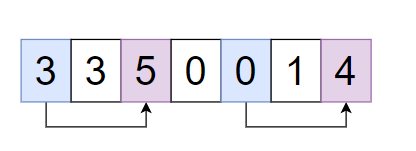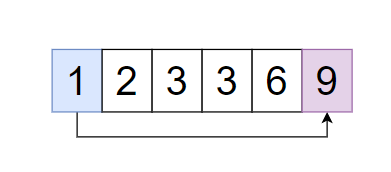2

Buy and Sell Stock - III

Difficulty: EASY
Avg. time to solve
10 min
Success Rate
90%

Problem Statement

You can perform a transition with these conditions -

1. Not allowed to engage in more than 1 transaction at a time, which means if you have bought stock then you can buy another stock before selling the first stock.

2. If you bought a stock at ‘X’ price and sold it at ‘Y’ price then the profits ‘Y - X’.
Note:
It is not compulsory to perform an exact '2' transaction.
Input format:
The first line of input contains an integer 'T' denoting the number of test cases.

The first line of each test case contains a single integer 'N', 'N' denotes the length of array ‘prices’.

The second line of each test case contains an 'N' space-separated integers, in which every integer denotes an element of the array "prices".
Output Format
For each test case, you need to print the maximum profit made by selling the stocks.
Note :
You do not need to print anything; it has already been taken care of.
Constraints:
1 <= T <= 50
1 <= N <= 10^4
0 <= A[i] <= 10^9

Time limit: 1 second
2
7
3 3 5 0 0 1 4
6
1 2 3 3 6 9
6
8
Explanation For Sample Input 1:
Test Case 1:Buy the first stock at ‘0-th’ day and sell at ‘2-nd’ day - ‘profit = 5 - 3 = 2’
Buy the second stock at ‘4’th day and sell at ‘6’th day - ‘profit = 4 - 0 = 4’
Total profit ‘2 + 4 = 6’.

Test Case 2:Buy the stock at ‘0’ day and sell at ‘5’ day - ‘profit = 9 - 1 = 8’.

Suppose we bought the first stock at ‘0-th ‘ day and sold at ‘2-nd’ then the profit will be ‘3 - 1 = 2’ and second stock at ‘3-rd’ day and sold at ‘5-th’ day then the profit will be ‘9 - 3 = 6’  so total profit ‘2 + 6 = 8’.
But there is no other possibility to get more profit than ‘8’.
2
5
1 2 4 3 2
4
9 8 7 6
Sample Output 2:
3
0Console